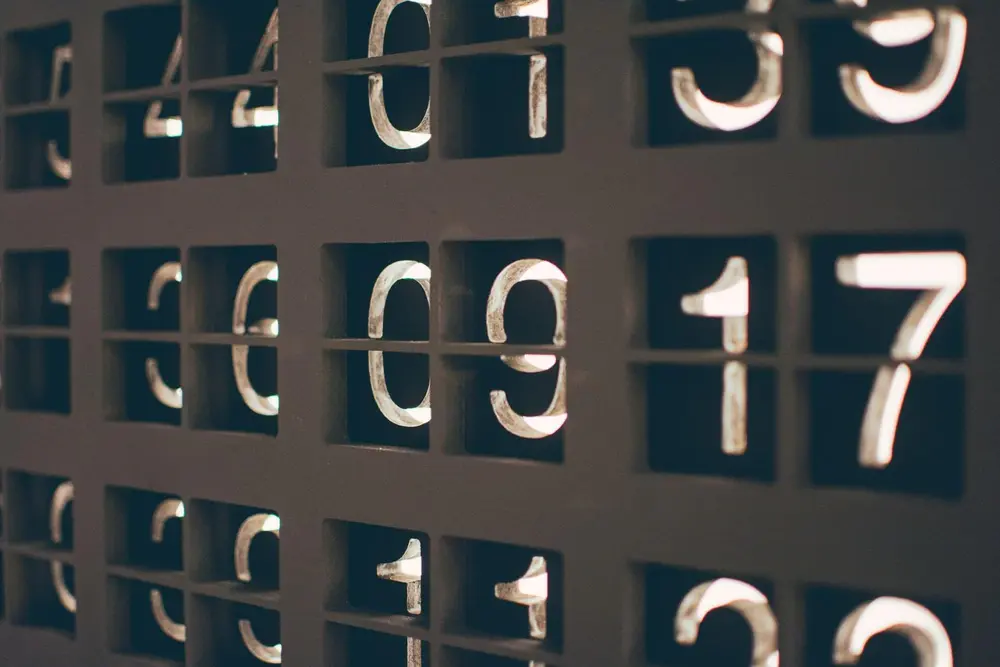# Ratios

#### Finding Ratios

• Finding a ratio between a set of numbers means finding out numerically how the numbers compare with each other.

#### For example

• Find the ratio between 50 pence and 1 pound.
• Put these into the same units: 1 pound is the same as 100 pence.
• Put them into the format of a ratio: 50 pence : 100 pence
• Simplify this to it's simplest form: 50 : 100 = 1 : 2
• This shows that the 1 pound was twice as much as the 50 pence.

#### Using Ratios

• A ratio shows the relation between each value. For a ratio a:b:c there are three different parts. Each of these represents a part of a whole. This ratio means there are a+b+c parts. This means a value which is to be split into the values with this ratio are in the parts a/(a+b+c) b/(a+b+c) and c/(a+b+c) which, together, make a whole.
• For example:
• Split 100 pounds in the ratio 3:2:5
• This means there are 10 parts, found by the sum of the values in the ratio. Each of the 10 parts are equal to each other. That means each part is 10 pounds, found by 100 divided by 10. We can then find how the 100 pounds is split:
• First part = ( 3 × 10 ) or ( 3/10 of 100 ) = 30 pounds
• Second part = ( 2 × 10 ) or ( 2/10 of 100 ) = 20 pounds
• Third part = ( 5 × 10 ) or ( 5/10 of 100 ) = 50 pounds
• This shows that 100 pounds split by the ratio - 3:2:5
• gives the values - 30 pounds : 20 pounds : 50 pounds
Number

Number

Number

Number

Number

Number